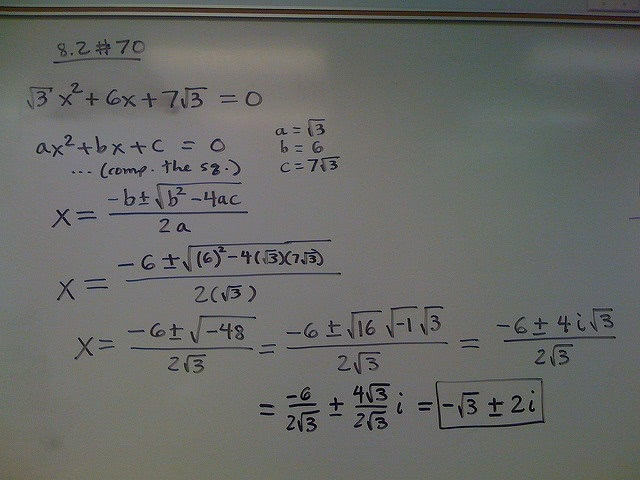How to Memorize Math Formulas for the ACTFlickr user Dan Bach

While the SAT will provide math formulas for you, the ACT will not—therefore, you’ll have to memorize the formulas yourself. This can make many students nervous, especially if math isn’t their strong suit. Fortunately there are ways to make memorization easier.

The likelihood of developing better recall is always higher when you are familiar with something. This principle stands true for math formulas required for ACT as well. It is pretty obvious that unless you have a phenomenal memory, you will not be able to recollect a formula you have seen just once.

The first step to memorizing formulas is to make sure that you at least recognize them when you see them. Make sure that you glance through the list of formulas and are cognizant of what they are when you see them. By itself, this won’t ensure that you remember the formula, but it is a start.

Several students tend to make the mistake of trying to memorize the formula without even knowing what it is, what it means, and why the formula is used. Reading the explanation or derivation of the formula can help simplify the process of memorizing the formula to a huge extent.

The brain forms memories by creating connections between various tidbits of information. The higher the number of connections your brain forms with the formula, the easier it is for your brain to retain said piece of information. Simply put, you have a better chance of recalling something the more you know about it.

Know the Test

You won’t use every mathematical formula known to man on the ACT, but you should know which ones you’ll require on the test.

There are 16 key math formulas you should know:

• Mean
• Probability
• Distance
• Slope
• Slope Intercept
• Midpoint
• Area of Triangle
• Area of Rectangle
• Area of Parallelogram
• Area of Circle
• Pythagorean Theorem
• Circumference
• Sine
• Cosine
• Tangent

Some formulas you’ll use more than others, but it’s still worthwhile to sit down and memorize all of them.

Mnemonic Devices

There are plenty of tricks when it comes to memorization. One of the most popular is the mnemonic device. Essentially, you create a rhyme, story, song, or acronym phrase that helps you recall information.

For example, our high school teacher taught us the Quadratic Formula by setting the instructions to the tune of “Pop Goes the Weasel.”

“X equals negative B, plus or minus the square root, of B squared minus 4 A C, all over 2 A!”

Practice

While the above tips can help simplify the process of memorizing formulas, they are not a replacement for actual practice. There is no running away from the fact that you will need to put hard work into solving problems using these formulas to ensure that you can recollect them when it matters the most.

While practicing, don’t try to verify the correctness of the formula until you finish solving the problem. Making mistakes helps you understand what you missed and why. If you keep glancing at the formula to check if you got it right, you will never get to the point where your brain is challenged sufficiently and forced to retain that information.

Use College Raptor to see how your ACT score affects your acceptance odds!